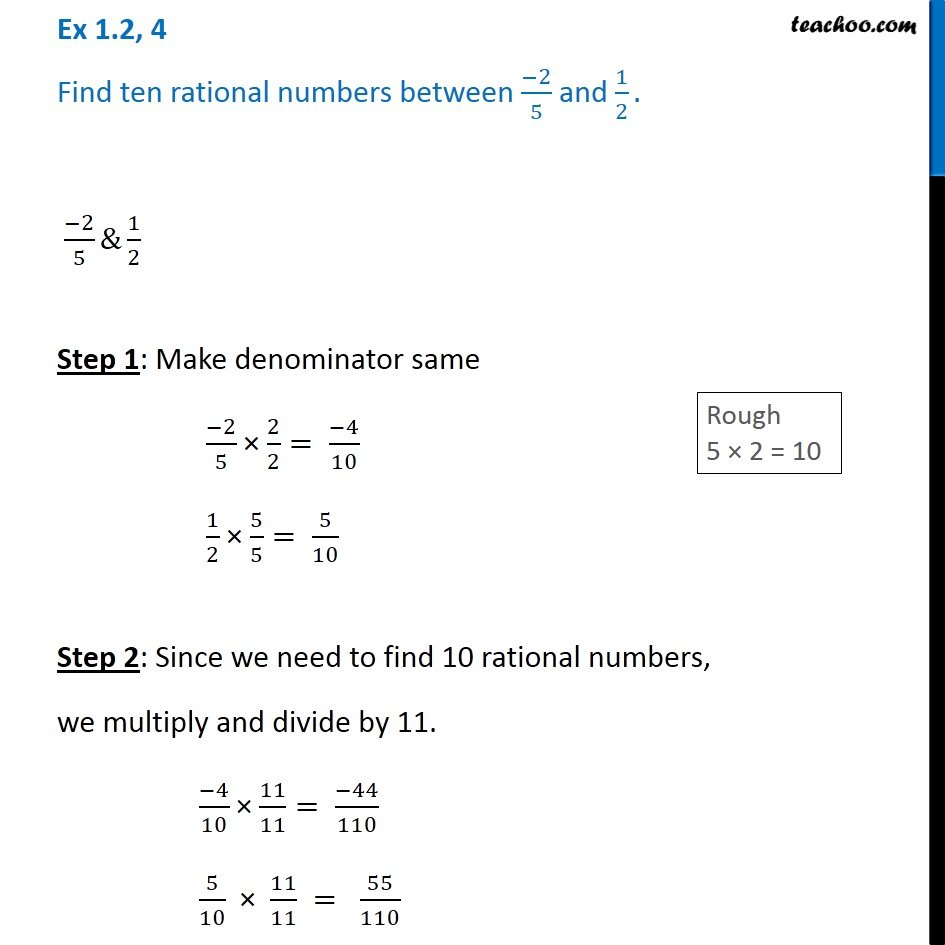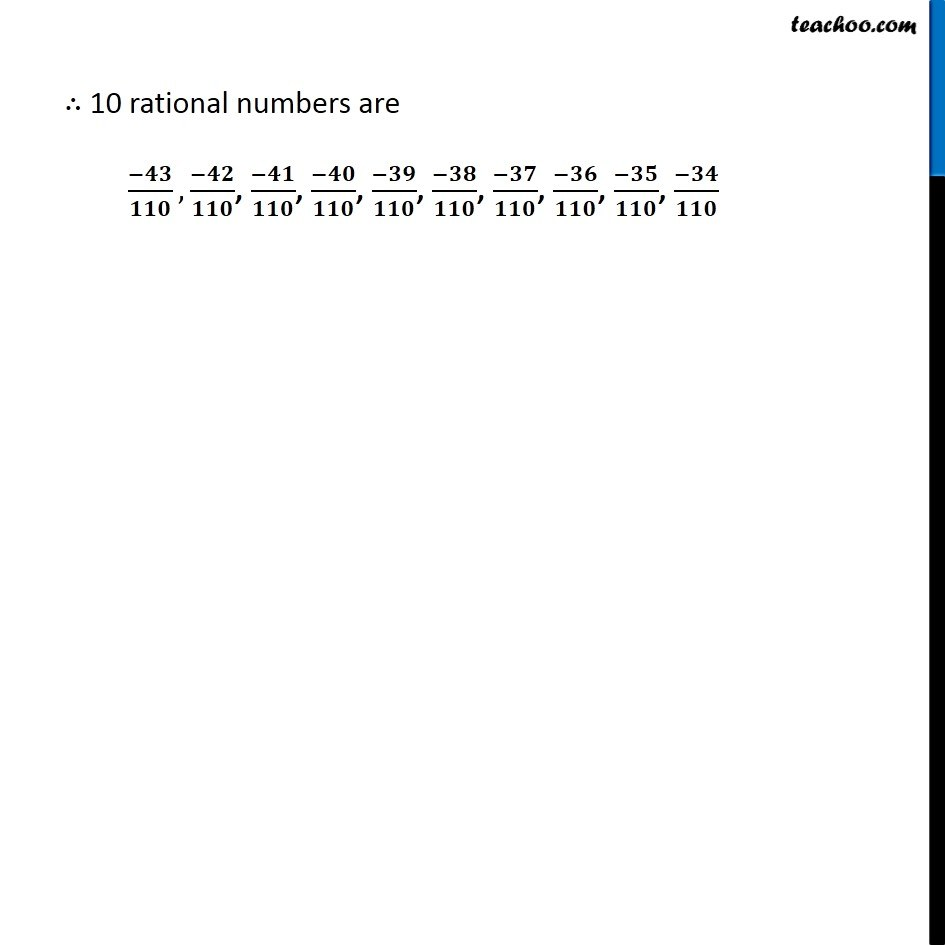Representing rational numbers on number line

Chapter 1 Class 8 Rational Numbers
Serial order wiseLearn in your speed, with individual attention - Teachoo Maths 1-on-1 Class

### Transcript

Question 4 Find ten rational numbers between -2/5 and 1/2 Step 1: Make denominator same Rough 5 × 2 = 10 (−2)/5 × 2/2= (−4)/10 1/2 × 5/5= 5/10 Step 2: Since we need to find 10 rational numbers, We multiply and divide by 11. (−4)/10 × 11/11= (−44)/110 5/10 × 11/11 = 55/110 ∴ 10 rational numbers are (−43)/110,(−42)/110, (−41)/110, (−40)/110, (−39)/110, (−38)/110, (−37)/110, (−36)/110, (−35)/110, (−34)/110